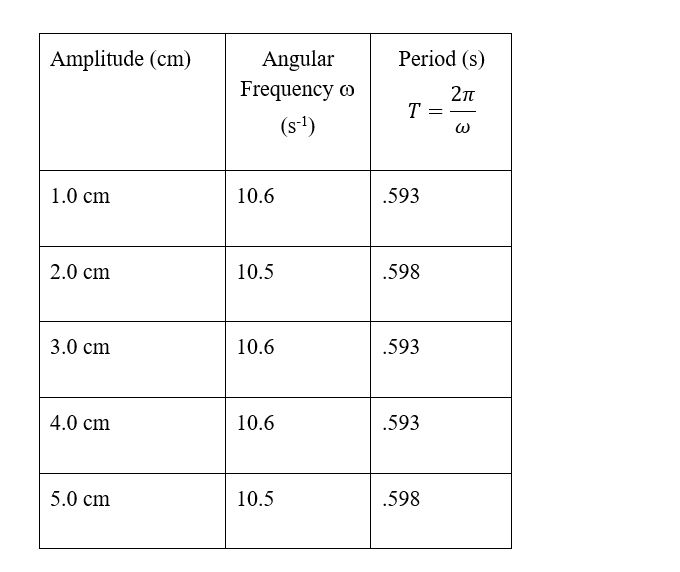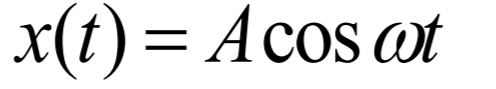# Problem: T = 2pi sqrt (m/k)Based on your data, does the amplitude of the motion effect the period? According to the formula for the period, should it?

###### FREE Expert Solution

For the given data set, the amplitude is increasing.

The period is constant despite the increase in amplitude.

90% (273 ratings)###### Problem DetailsT = 2pi sqrt (m/k)

Based on your data, does the amplitude of the motion effect the period? According to the formula for the period, should it?